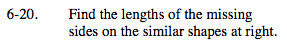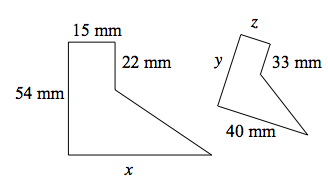### Home > MC2 > Chapter 6 > Lesson 6.1.2 > Problem6-20

6-20.Similar shapes have sides with equal ratios to each other. To find each unknown side, compare its ratio to a known side.For example, the ratio of the 15 mm side to the 22 mm side should be equal to the ratio of the z side to the 33 mm side.

$\frac{15}{22} = \frac{z}{33}$

Now solve for z.

z = 22.5 mm

You can now use that to find ratios for the other unknown sides.

Write two more ratios to solve for side x and side y.

$\frac{15}{54} = \frac{22.5}{y}$

15 y = (22.5) (54)

y = 81 mm
Now what does x equal?

Explore the problem with the etool below.
Click the link at right for the full version of the eTool: MC2 6-20 HW eTool